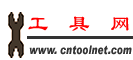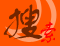工具搜索引擎 加入收藏 意见反馈 帮助中心 繁體中文 资讯标题 资讯内容 商业机会 商机简介 公司名称 公司简介 超市名称 超市简介
 首页 工具超市 产品信息 资讯中心 工具黄页 站长工具 实用工具 会员管理 联系我们

数据类型中整数和浮点数的使用

常见的整型数学运算

`Private Sub btnIntMath_Click(ByVal sender As System.Object, _`
`ByVal e As System.EventArgs) Handles btnIntMath.Click`
` `
`End Sub`

`Private Sub btnIntMath_Click(ByVal sender As System.Object, _`
`ByVal e As System.EventArgs) Handles btnIntMath.Click`
`  'Declare variable`
`  Dim intNumber As Integer`
` `
`  'Set number, add numbers, and display results`
`  intNumber = 16`
`  intNumber = intNumber + 8`
`  MessageBox.Show("Addition test... " & intNumber, "Integer Math")`
` `
`  'Set number, subtract numbers, and display results`
`  intNumber = 24`
`  intNumber = intNumber - 2`
`  MessageBox.Show("Subtraction test... " & intNumber, "Integer Math")`
` `
`  'Set number, multiply numbers, and display results`
`  intNumber = 6`
`  intNumber = intNumber * 10`
`  MessageBox.Show("Multiplication test... " & intNumber, "Integer Math")`
` `
`  'Set number, divide numbers, and display results`
`  intNumber = 12`
`  intNumber = intNumber / 6`
`  MessageBox.Show("Division test... " & intNumber, "Integer Math")`
` `
`End Sub`

`  intNumber = 16`
`  intNumber = intNumber + 8`
`  MessageBox.Show("Addition test... " & intNumber, "Integer Math")`

Visual Basic 2005 中的整数类型 (Integer) 能表示 -2,147,483,648 到 2,147,483,647 之间的整数。

简写运算符

`intNumber = intNumber + 8`

`intNumber += 8`

`intNumber -= 8`
`intNumber *= 8`
`intNumber /= 8`

整形运算的问题

`'Try multiplying numbers...`
`intNumber = 6`
`intNumber = intNumber * 10.23`

`'Try dividing numbers...`
`intNumber = 12`
`intNumber = intNumber / 5`

12 除以 5 应该等于 2.4，但是 intNumber 的返回值却是 2。

浮点运算

`Private Sub btnFloatMath_Click(ByVal sender As System.Object, _`
`ByVal e As System.EventArgs) Handles btnFloatMath.Click`
` `
`End Sub`

`Private Sub btnFloatMath_Click(ByVal sender As System.Object, _`
`ByVal e As System.EventArgs) Handles btnFloatMath.Click`
`  'Declare variable`
`  Dim dblNumber As Double`
` `
`  'Set number, multiply numbers, and display results`
`  dblNumber = 45.34`
`  dblNumber *= 4.333`
`  MessageBox.Show("Multiplication test... " & dblNumber, "Floating Points")`
` `
`  'Set number, divide numbers, and display results`
`  dblNumber = 12`
`  dblNumber /= 7`
`  MessageBox.Show("Division test... " & dblNumber, "Floating Points")`
`End Sub`

双精度浮点数和单精度浮点数

VB 2005 入门教程

If...Then...Else 条件语句
Select...Case 条件语句
For...Next 循环语句
 暂无相关评论

 发表评论： 用户: 验证码

 版权所有 海力工具网 电话：0523-86864621